# Volume of revolution around y-axis....stumped

• Kerrigoth
In summary, the area between the straight line and the parabola is revolved around the y-axis. The volume of revolution is

## Homework Statement

The total area between a straight line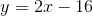and the parabola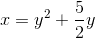is revolved around the y-axis. What is the volume of revolution?

According to the book, the answer is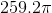; My answer comes out to be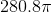## Homework Equations

1.2.## The Attempt at a Solution

1. Rewrite the second equation in the form of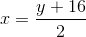2. Set the two equations to each other and find which values of 'y' they intercept.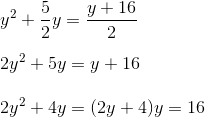This yields the functions intercept at y= -4 and y=2

3. The cylindrical shells method will be used to find the volume of revolution around the y-axis. My equation is set up to be.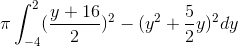4. I used Wolfram to save a bunch of Tex...my answer comes out to beI must be approaching the problem in a wrong way but am stumped figuring out why. Any help would be greatly appreciated.

#### Attachments

Kerrigoth said:
1. Rewrite the second equation in the form of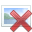I would take a second look at what you're doing...

Your graph has both positive and negative x-values in the intersecting area, you are double-counting the corresponding volume of rotation there.
Replusz said:
I would take a second look at what you're doing...
That is fine.

mfb said:
Your graph has both positive and negative x-values in the intersecting area, you are double-counting the corresponding volume of rotation there.That is fine.

original graph: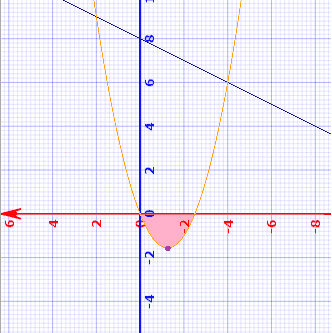I see the problem the pointed out. (I shaded the double counted area pink)

The only thing I can think of so far is to shift the graph upwards so there are no negative regions. I thought I'd try:
1. Find the minimum of ##x=y^2+\frac{5}{2}y##
##\frac{\mathrm{d} }{\mathrm{dy} }(y^2+\frac{5}{2}y)=2y+\frac{5}{2}=0## when ##y=-\frac{5}{4}##

This means the minimum is at ##(-\frac{25}{16},-\frac{5}{4})##

2. Shift the graph upwards by adding ##\frac{25}{16}## value to both equations.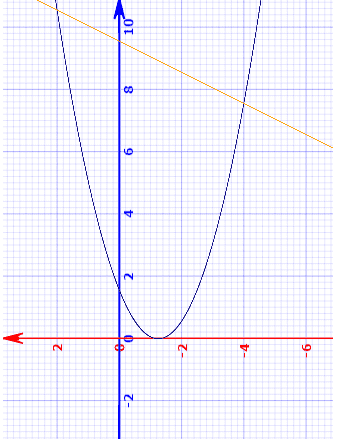3. Redo the integration
##\pi\int_{-4}^{2}(\frac{y+16}{2} +\frac{25}{16})^2 - (y^2+\frac{5y}{2}{}+\frac{25}{16})^2 dy##

But I end up with another wrong answer. ##393.3\pi##

#### Attachments

No, shifting doesn't work, because you multiply by the (now shifted) value in the integral.

You can subtract the double-counted component separately.

mfb said:
No, shifting doesn't work, because you multiply by the (now shifted) value in the integral.

You can subtract the double-counted component separately.

Ok, so I'll try that.

(again the relevant area whose volume will be counted twice is shaded in pink)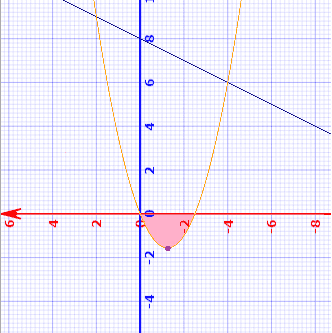So the area I have which includes the double counted volume is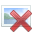which comes fromNow I want to subtract the volume (which comes from the area shaded pink)

1. First find the values which satisfy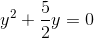...they come out to be 0 and ##-\frac{5}{2}##
2. Find the volume when this area is rotated around the y-axis
##\pi\int_{-5/2}^{0}y^2+\frac{5}{2}y\ dy## which comes out to be ##3.2552\pi##
3. Subtract this volume from the original volume to eliminate double counted volume
##280.8\pi -3.2552\pi = 277.545\pi##

Your recommendation to eliminate the double counted volume makes sense to me but the answer,##277.545\pi## , still falls short of the book's.I think that you might not be being careful enough keeping track of which axis is which. In the graph above, which one is the x-axis? Which is the y-axis?

The equations that form the boundaries are y = 2x - 16 and ##x = y^2 + (5/2)y##.

On your graph, the positive y-axis actually goes off to the left.

Mark44 said:I think that you might not be being careful enough keeping track of which axis is which. In the graph above, which one is the x-axis? Which is the y-axis?

The equations that form the boundaries are y = 2x - 16 and ##x = y^2 + (5/2)y##.

On your graph, the positive y-axis actually goes off to the left.

The pictures provided are normal graphs rotated 90 degrees counter clockwise. The red is the y-axis and the blue is the x-axis.

I should have labelled the graph better but can assure they are still correct. I rotated the picture after generating to ease visualization for myself.

kerrigoth said:
2. Find the volume when this area is rotated around the y-axis
##\pi\int_{-5/2}^{0}y^2+\frac{5}{2}y\ dy## which comes out to be ##3.2552\pi##
Your integral isn't right. The typical volume element is ##\pi x^2 \Delta y##, but ##x= y^2 + (5/2)y##. You're not squaring the radius (x).

Mark44 said:
Your integral isn't right. The typical volume element is ##\pi x^2 \Delta y##, but ##x= y^2 + (5/2)y##. You're not squaring the radius (x).

My sincerest apologies. I did square it when calculating but mistyped the Tex.

Nonetheless, ##\pi\int_{-5/2}^{0}(y^2+\frac{5}{2}y)^2\ dy## does equal ##3.2552\pi##. Being said, my final answer ends up being ##277.545\pi## which is ##18.346\pi## larger than the book's answer.

OK, I think I have this figured out. Your first integral is incorrect -- ##\pi \int_{-4}^2(\frac{y + 16}{2})^2 dy##

This is harder to see because the graph you posted is rotated. In the unrotated graph, the integral above doesn't represent the volume of rotation of the region bounded by the parabola, the y-axis, and the line y = 2x - 16. If you use horizontal slices, and revolve them around the y-axis, the right ends of all of these slices end on the line; i.e., with x = (y + 16)/2. However, your integral above assumes that the left ends of these slices end at the y-axis; i.e., with x = 0. This isn't so. If y > 0 or if y < -5/2, the left ends of these slices are on the parabola.

This means that you will need three different integrals to get the volume when the region bounded by the parabola, the line, and the y-axis is revolved.

Your integral overestimates the actual area, so using three integrals instead of the one you show should give a result that agrees with the book's answer or is at least closer to it.

Mark44 said:
However, your integral above assumes that the left ends of these slices end at the y-axis; i.e., with x = 0. This isn't so. If y > 0 or if y < -5/2, the left ends of these slices are on the parabola.

This means that you will need three different integrals to get the volume when the region bounded by the parabola, the line, and the y-axis is revolved.

Your integral overestimates the actual area, so using three integrals instead of the one you show should give a result that agrees with the book's answer or is at least closer to it.

This is my first integral.Yes, the integral above represents the volume generated by the area between the line and the parabola.

But then I go on to subtract the volume generated by the area of the parabola between y > 0 or if y < -5/2 with the following integral:

##\pi\int_{-5/2}^{0}(y^2+\frac{5}{2}y)^2\ dy##

Which should eliminate the overestimated area

Kerrigoth said:
This is my first integral.Yes, the integral above represents the volume generated by the area between the line and the parabola.

But then I go on to subtract the volume generated by the area of the parabola between y > 0 or if y < -5/2
What you mean is the region of the parabola with -5/2 < y < 0. That interval on the y-axis includes the part of the parabola that includes the vertex, and is the part that is to the left of the y-axis.
Kerrigoth said:
with the following integral:

##\pi\int_{-5/2}^{0}(y^2+\frac{5}{2}y)^2\ dy##

Which should eliminate the overestimated area
I don't see why that wouldn't work. Are you still getting a larger value than is given as the answer? If so, and all your work is correct, it might mean the book's answer is wrong. It has been known to happen.

I calculated it from scratch using a different approach (start with the cone and then subtract the slices due to the parabolas), and I get 284.055 pi (exact value 272693/960 pi).

@Kerrigoth: In post #6, you should add the 3.255 pi, as your initial approach subtracted it twice, so in post #6 you actually subtracted it three times then instead of once. If you add it instead if subtract it, you get the same result as I got.